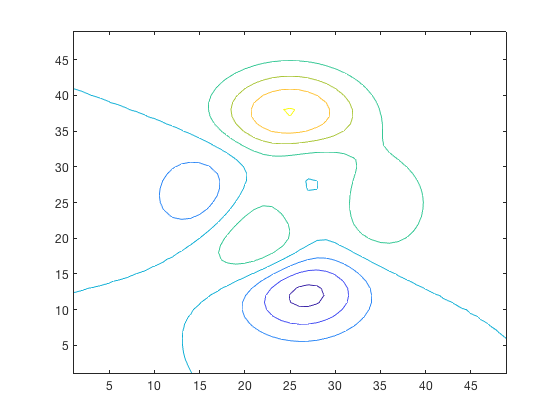# C2xyz documentation

C2XYZ converts a contour matrix (as returned by the contour function) into x, y, and corresponding z coordinates.

## Syntax

```[x,y] = C2xyz(C)
[x,y,z] = C2xyz(C)```

## Description

[x,y] = C2xyz(C) returns x and y cell arrays of coordinates of contours in a contour matrix C.

[x,y,z] = C2xyz(C) also returns corresponding z values as double.

## Example

Given a contour plot, you want to know the (x,y) coordinates of the contours, as well as the z value corresponding to each contour line.

```C = contour(peaks);
[x,y,z] = C2xyz(C);
```This returns 1 x numberOfContourLines cells of x values and y values, and their corresponding z values are given in a 1 x numberOfContourLines array. Let's pick out all the x, y locations where z = 0, and make that contour line a heavy black line:

```hold on; % Allows plotting atop the preexisting peaks plot.

for n = find(z==0) % only loop through the z = 0 values.
plot(x{n},y{n},'k','linewidth',2)
end
```And let's make all the z = -2 lines red and dotted:

```for n = find(z==-2) % now loop through the z = -2 values.
plot(x{n},y{n},'r:','linewidth',2)
end
```## Author Info

Created by Chad Greene, of the University of Texas Institute for Geophysics, August 2013. Updated August 2014 for inclusion in Antarctic Mapping Tools for Matlab (Greene et al., 2017), and updated again in 2019 for inclusion in the Climate Data Toolbox for Matlab.